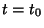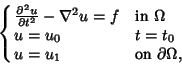## Initial Value Problem

An initial value problem is a problem that has its conditions specified at some time. Usually, the problem is an Ordinary Differential Equation or a Partial Differential Equation. For example,wheredenotes the boundary of, is an initial value problem.